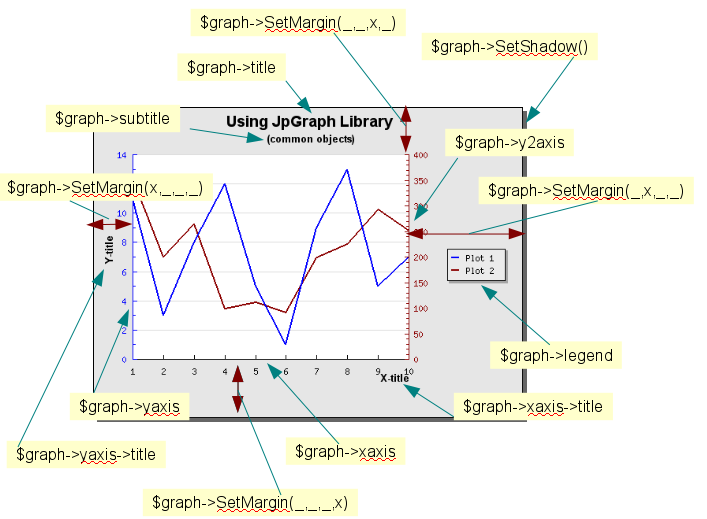## Chapter 12. Commonalities for all graphs

All graph scripts follow, to some extent, the same structure. All scripts must start by creating an instance of the Graph class. The Graph class represent the entire graph and can have one or more plots build up from the data series. Almost all methods that are used to control the appearance of the graph are methods of this class.

In order to give a feel for common objects we show examples of two of the most commonly used graph types, cartesian graphs and pie graphs in the following two sections.

## 12.1. Common objects for cartesian graphs (x-, y-graphs)

In all documentation of the library the convention is that the instance of the Graph class will be stored in a variable named "`\$graph`". Most of the parts that make up a graph are available as instance variables of the Graph class. In order to illustrate some of the commonly used instance variables Figure 12.1 shows a basic graph with a number of the objects that can be manipulated in the graph scripts.

Figure 12.1. Commonly used objects in a graph (`common-obj-graph.php`)We note a few things in Figure 12.1

• All graphs will start by including the necessary library files via one or more "require_once" PHP statements

• All graph scripts (for 2D linear type graphs) will have the following two method calls

 ```1 2 ``` ```\$graph = new Graph(\$width, \$height); \$graph->SetScale('...');```

These two calls create the necessary instance of the core Graph class that represents the entire graph and specifies what scale should be used for the y- and x-axis. In Figure 12.1 we are also using a second -axis so in this case the script will also contain a call to

 ```1 ``` `\$graph->SetY2Scale('...');`

• Most graphs will also adjust the left, right, top and bottom margin. In Figure 12.1 the margins are shown with red arrows. The margins are specified with the method

 ```1 ``` `\$graph->SetMargin(\$left,\$right,\$top,\$bottom);`

• All text objects (e.g. graph and axis titles) are instances of the common Text class. This means that the text, font, color are specified in the same way. For example the code to set the title and subtitle in the graph above is

 ```1 2 3 4 5 6 ``` ```\$graph->title->SetFont(FF_ARIAL, FS_BOLD, 14); \$graph->title->Set("Using JpGraph Library"); \$graph->title->SetMargin(10); \$graph->subtitle->SetFont(FF_ARIAL, FS_BOLD, 10); \$graph->subtitle->Set('(common objects)');```

In a similar way the code to set the titles of the axis are

 ```1 2 3 4 5 ``` ```\$graph->xaxis->title->SetFont(FF_ARIAL, FS_BOLD, 10); \$graph->xaxis->title->Set("X-title"); \$graph->yaxis->title->SetFont(FF_ARIAL, FS_BOLD, 10); \$graph->yaxis->title->Set("Y-title");```

• The graph uses a legend in two rows to name each data series. The position of the legend is controlled by the script and in Figure 12.1 we placed it on the right side. It is also possible to in detail to adjust the layout of the names in the data series. For example we could change the layout of the legend to position all the names of the data series in one row as opposed to one column which is the default. The number of columns and/or rows is user settable.

• Finally all graphs will end with a call to `Stroke()` or one of its variants (`StrokeCSIM()`, `StrokeCSIMIMage()`). As was described in Part II this will send back the constructed image to the client, usually a browser.

For other types of graphs (for example Gantt charts) some of the above standards still apply but since they have a very different usage and layout the instance variables will be different.